# 19.4 Equipotential lines  (Page 2/4)

 Page 2 / 4

## Grounding

A conductor can be fixed at zero volts by connecting it to the earth with a good conductor—a process called grounding.

Because a conductor is an equipotential, it can replace any equipotential surface. For example, in [link] a charged spherical conductor can replace the point charge, and the electric field and potential surfaces outside of it will be unchanged, confirming the contention that a spherical charge distribution is equivalent to a point charge at its center.

[link] shows the electric field and equipotential lines for two equal and opposite charges. Given the electric field lines, the equipotential lines can be drawn simply by making them perpendicular to the electric field lines. Conversely, given the equipotential lines, as in [link] (a), the electric field lines can be drawn by making them perpendicular to the equipotentials, as in [link] (b).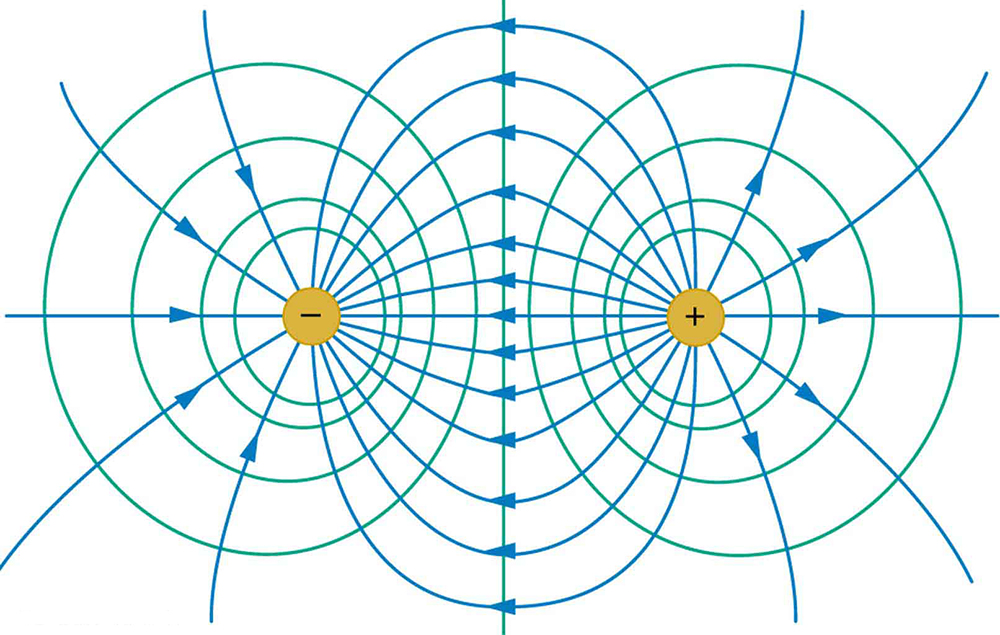The electric field lines and equipotential lines for two equal but opposite charges. The equipotential lines can be drawn by making them perpendicular to the electric field lines, if those are known. Note that the potential is greatest (most positive) near the positive charge and least (most negative) near the negative charge.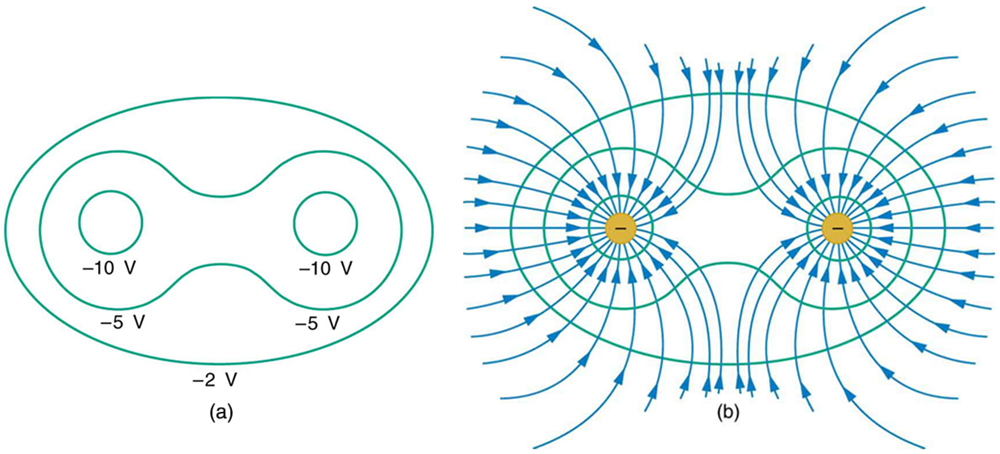(a) These equipotential lines might be measured with a voltmeter in a laboratory experiment. (b) The corresponding electric field lines are found by drawing them perpendicular to the equipotentials. Note that these fields are consistent with two equal negative charges.

One of the most important cases is that of the familiar parallel conducting plates shown in [link] . Between the plates, the equipotentials are evenly spaced and parallel. The same field could be maintained by placing conducting plates at the equipotential lines at the potentials shown.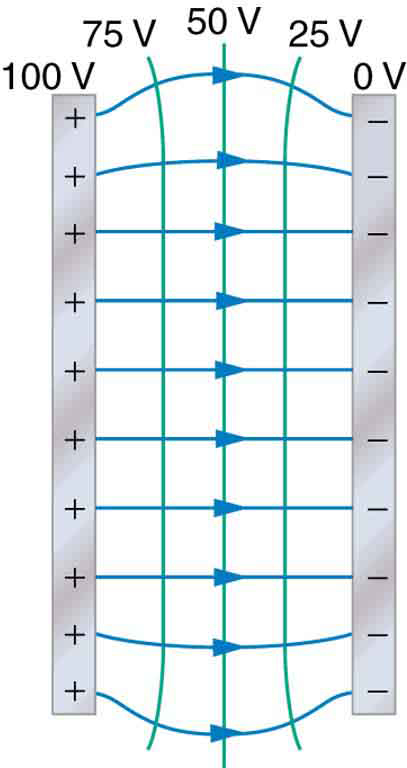The electric field and equipotential lines between two metal plates.

An important application of electric fields and equipotential lines involves the heart. The heart relies on electrical signals to maintain its rhythm. The movement of electrical signals causes the chambers of the heart to contract and relax. When a person has a heart attack, the movement of these electrical signals may be disturbed. An artificial pacemaker and a defibrillator can be used to initiate the rhythm of electrical signals. The equipotential lines around the heart, the thoracic region, and the axis of the heart are useful ways of monitoring the structure and functions of the heart. An electrocardiogram (ECG) measures the small electric signals being generated during the activity of the heart. More about the relationship between electric fields and the heart is discussed in Energy Stored in Capacitors .

## Phet explorations: charges and fields

Move point charges around on the playing field and then view the electric field, voltages, equipotential lines, and more. It's colorful, it's dynamic, it's free.

## Section summary

• An equipotential line is a line along which the electric potential is constant.
• An equipotential surface is a three-dimensional version of equipotential lines.
• Equipotential lines are always perpendicular to electric field lines.
• The process by which a conductor can be fixed at zero volts by connecting it to the earth with a good conductor is called grounding.

## Conceptual questions

What is an equipotential line? What is an equipotential surface?

Explain in your own words why equipotential lines and surfaces must be perpendicular to electric field lines.

Can different equipotential lines cross? Explain.

## Problems&Exercises

(a) Sketch the equipotential lines near a point charge + $q$ . Indicate the direction of increasing potential. (b) Do the same for a point charge $–3\phantom{\rule{0.25em}{0ex}}q$ .

Sketch the equipotential lines for the two equal positive charges shown in [link] . Indicate the direction of increasing potential.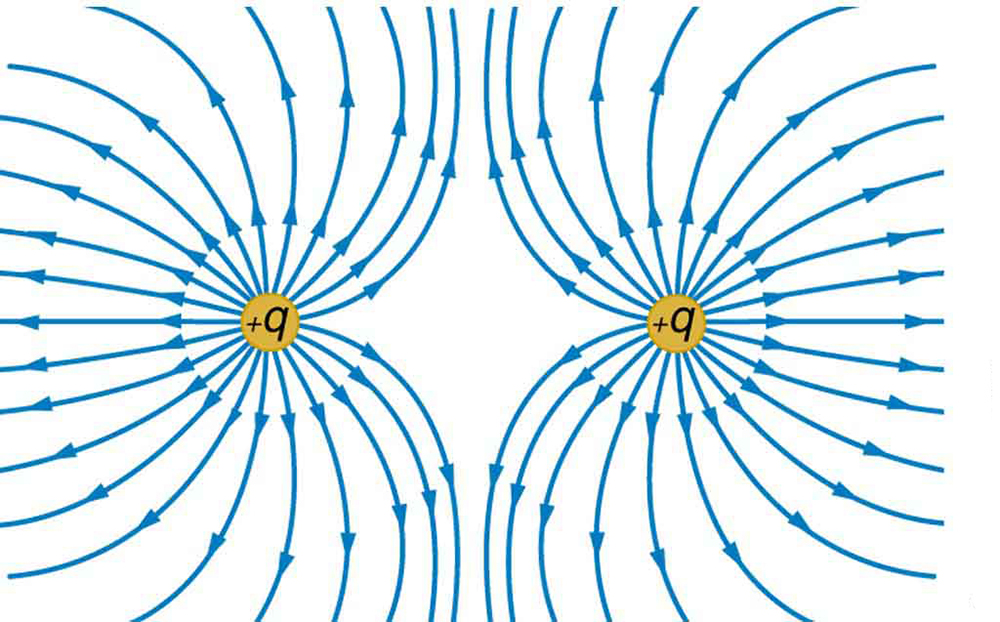The electric field near two equal positive charges is directed away from each of the charges.

[link] shows the electric field lines near two charges ${q}_{1}$ and ${q}_{2}$ , the first having a magnitude four times that of the second. Sketch the equipotential lines for these two charges, and indicate the direction of increasing potential.

Sketch the equipotential lines a long distance from the charges shown in [link] . Indicate the direction of increasing potential.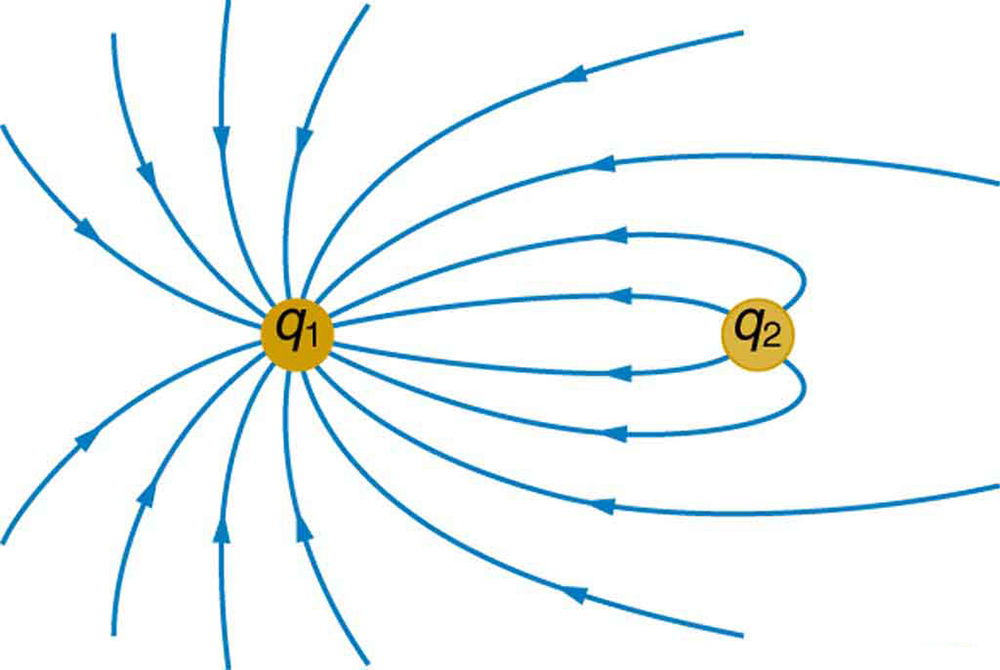The electric field near two charges.

Sketch the equipotential lines in the vicinity of two opposite charges, where the negative charge is three times as great in magnitude as the positive. See [link] for a similar situation. Indicate the direction of increasing potential.

Sketch the equipotential lines in the vicinity of the negatively charged conductor in [link] . How will these equipotentials look a long distance from the object?

Sketch the equipotential lines surrounding the two conducting plates shown in [link] , given the top plate is positive and the bottom plate has an equal amount of negative charge. Be certain to indicate the distribution of charge on the plates. Is the field strongest where the plates are closest? Why should it be?

(a) Sketch the electric field lines in the vicinity of the charged insulator in [link] . Note its non-uniform charge distribution. (b) Sketch equipotential lines surrounding the insulator. Indicate the direction of increasing potential.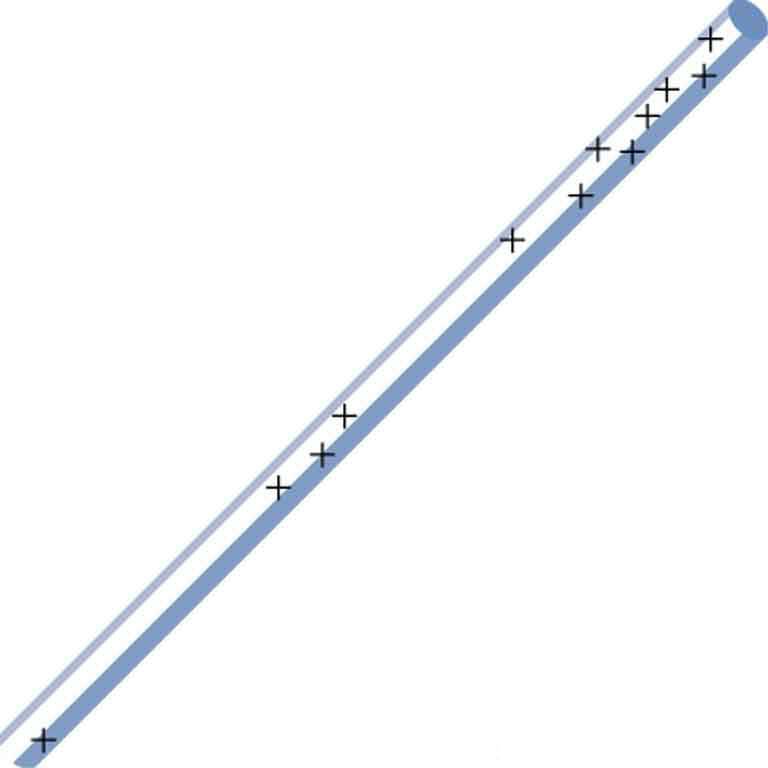A charged insulating rod such as might be used in a classroom demonstration.

The naturally occurring charge on the ground on a fine day out in the open country is $\text{–1}\text{.}\text{00}\phantom{\rule{0.25em}{0ex}}{\text{nC/m}}^{2}$ . (a) What is the electric field relative to ground at a height of 3.00 m? (b) Calculate the electric potential at this height. (c) Sketch electric field and equipotential lines for this scenario.

The lesser electric ray ( Narcine bancroftii ) maintains an incredible charge on its head and a charge equal in magnitude but opposite in sign on its tail ( [link] ). (a) Sketch the equipotential lines surrounding the ray. (b) Sketch the equipotentials when the ray is near a ship with a conducting surface. (c) How could this charge distribution be of use to the ray?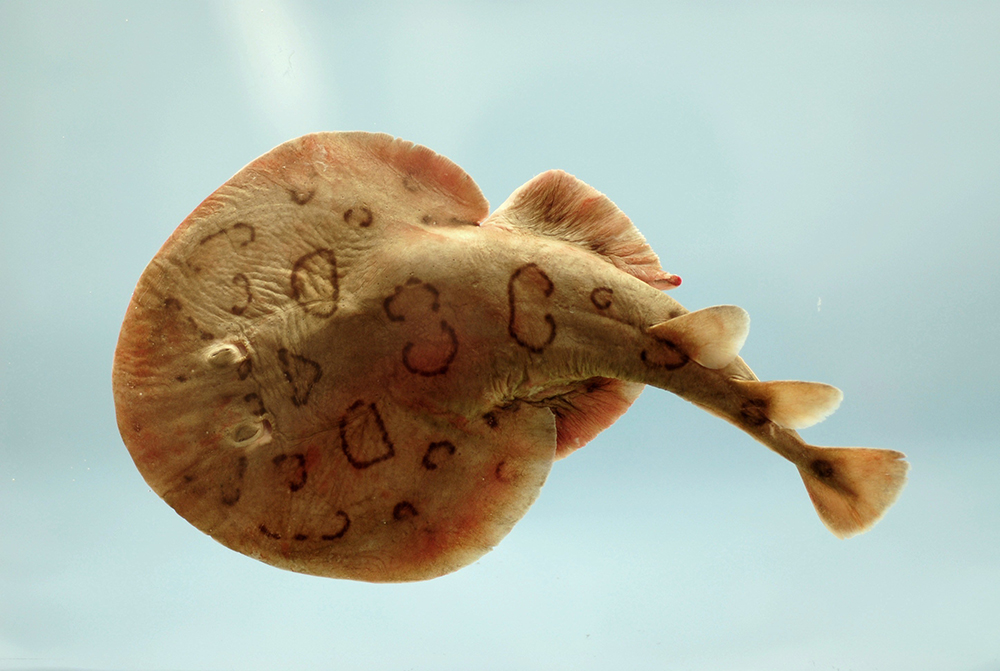Lesser electric ray ( Narcine bancroftii ) (credit: National Oceanic and Atmospheric Administration, NOAA's Fisheries Collection).

#### Questions & Answers

what is torque
The turning effect of force is called torque.
Uzair
what there factors affect the surface tension of a liquid
formula for impedance
ehat is central forces
what is distance?
What does mean ohms law imply
ohms law state that the electricity passing through a metallic conductor is directly proportional to the potential difference across its end
muyiwa
what is matter
Anything that occupies space
Kevin
Any thing that has weight and occupies space
Victoria
Anything which we can feel by any of our 5 sense organs
Suraj
Right
Roben
thanks
Suraj
what is a sulphate
Alo
Alo
the time rate of increase in velocity is called
acceleration
Emma
What is uniform velocity
Victoria
Greetings,users of that wonderful app.
how to solve pressure?
how do we calculate weight and eara eg an elefant that weight 2000kg has four fits or legs search of surface eara is 0.1m2(1metre square) incontact with the ground=10m2(g =10m2)
Cruz
P=F/A
Mira
can someone derive the formula a little bit deeper?
Bern
what is coplanar force?
forces acting and lying on d same plane
Promise
what is accuracy and precision
How does a current follow?
follow?
akif
which one dc or ac current.
akif
how does a current following?
Vineeta
?
akif
AC current
Vineeta
AC current follows due to changing electric field and magnetic field.
akif
you guys are just saying follow is flow not follow please
Abubakar
ok bro thanks
akif
flows
Abubakar
but i wanted to understand him/her in his own language
akif
but I think the statement is written in English not any other language
Abubakar
my mean that in which form he/she written this,will understand better in this form, i write.
akif
ok
Abubakar
ok thanks bro. my mistake
Vineeta
u are welcome
Abubakar
what is a semiconductor
substances having lower forbidden gap between valence band and conduction band
akif
what is a conductor?
Vineeta
replace lower by higher only
akif
convert 56°c to kelvin
Abubakar
How does a current follow?
Vineeta
A semiconductor is any material whose conduction lies between that of a conductor and an insulator.
AKOWUAH
what is Atom? what is molecules? what is ions?
atoms are the smallest unit of an element which is capable of behaving as a single unit
Promise
a molecule is d smallest unit of a substances capable of independent existence and can also retain the chemical proper ties of that substance
Promise
an ion is referred to as freely moving charged particles
PromiseByBy Jordon HumphreysBy Sam LuongBy Nick SwainBy